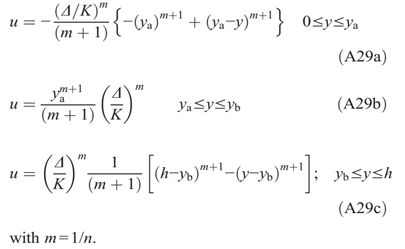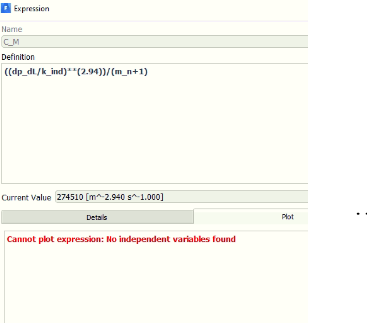ecrod
SubscriberAbove to below are u_a, u_p and u_b. All of them have the same multiplier parameter as function of pressure gradient ((DELTA/K)^m)/(m+1). I beleive that K [Pa.s^0.34] can be a cause to velocity as [m s^-1.000]. Here are the units of this term ((DELTA/K)^m)/(m+1).nnn